|

# 销量破2亿,袁姗姗直播助力TCL618狂欢趴

618活动预热持续升温，TCL在6月1日迎来开门红后，各项销售数据持续刷新，不断攀登新高。目前最新数据显示，6月1日至2日两天，全网销售额已突破2亿。

作为TCL品牌季大使，拥有“马甲线女神”称号的袁姗姗于6月2日晚上8点，在TCL京东直播间、一直播等直播平台与广大网友进行了一场直播互动，和TCL酷友科技CEO单晓鹏一起，为观众分享了多款TCL品质健康生活神器。现场最高在线峰值将近50万人，点赞493.6万次，截止目前总观看量达到550万次。这次直播，单晓鹏亲自担任主持人。作为TCL酷友科技CEO，年轻帅气的单晓鹏在直播刚开始就自报花名“鹏少”，瞬间得到广大网友的互动点赞认可。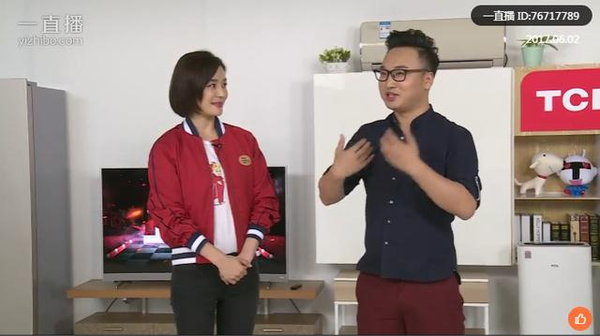（直播现场，袁姗姗和TCL酷友科技CEO单晓鹏愉快互动）

提到袁姗姗，大家第一反应都是希望能拥有和她一样的马甲线。单晓鹏自然不会错过此次良机，现场发问：如何能拥有像女神这般的马甲线身材？姗姗也不吝赐教，大方介绍自己的健身秘诀。同时，姗姗也提到自己健身的衣物，尤其是在南方梅雨季节清洗烘干的困扰。此时，单晓鹏向女神隆重推荐了TCL的变频洗烘一体机TCL XQG85-F14303HBDP，完美解决了女神的难题。这台洗衣机拥有双大容量，8.5公斤洗涤+5公斤烘干，40分钟就能完成洗涤+烘干的任务，无需晾晒，即洗即穿。不仅如此，在洗涤时还能做到超稳定静音，安静无打扰。

让人意外的是，单晓鹏现场居然打开洗衣机，并在运转中的洗衣机上搭好积木，即兴和姗姗玩起了抽积木的游戏，不亦乐乎。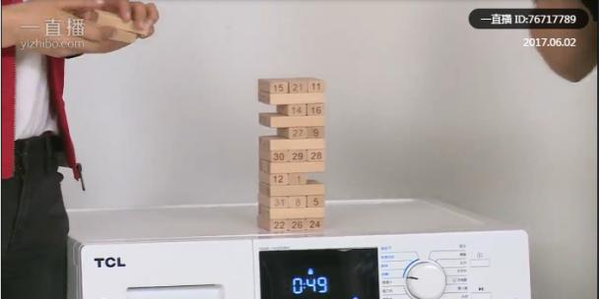（袁姗姗和单晓鹏在开启运作的TCL洗衣机旁玩抽积木游戏，TCL洗衣机稳定又静音）游戏结束，单晓鹏又向姗姗介绍了一款源于意大利美学设计的超高颜值TCL罗马柱空调TCL KFRd-51LW/RX11BpA，作为一款超一级智能全直流变频空调，950mn大风口，开机噪音低至19分贝，可安静享受清凉好空气。堪比工艺品的完美线条设计很快就俘获了女神芳心，让女神爱不释手。（TCL酷友科技CEO单晓鹏为袁姗姗介绍美观又节能的罗马柱空调）姗姗表示，自己经常熬夜拍戏且睡眠很轻，目前炎热的天气又离不开空调的使用。当单晓鹏提到此款空调不仅颜值高，耗电少，而且超级静音的时候，姗姗现场种草，直接提出能否抱回家。

热爱工作，更追求品质生活

作为一位广受欢迎的明星大咖，袁姗姗每天的工作异常繁忙，再加上她对自己的高要求以及事事求精的工作态度，为了保证自己有充沛的精力来面对工作上的繁忙，姗姗对自己的健身运动和饮食健康极度重视。

说到这些方面，单晓鹏非常认同。TCL酷友科技CEO单晓鹏每天虽然会花大量的时间在工作上，但同样很关注自己的健康和饮食。抓住这次机会，单晓鹏现场向姗姗请教她的健身秘诀和独家食材配方。

作为交换，单晓鹏又向姗姗推荐了一款TCL BCD-499WEF1冰箱。这款冰箱拥有超大容量，18个独立分区能把所有健康美食通通装进冰箱。更重要的是，冰箱自带的智慧摆风功能，左右均匀制冷，能让食物保鲜更持久。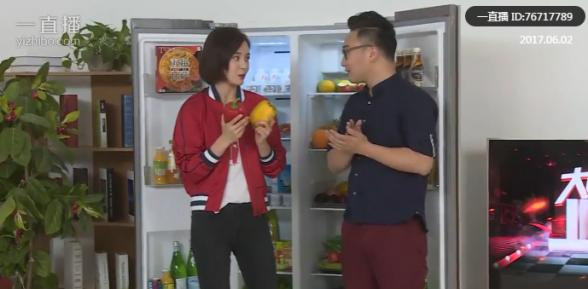（袁姗姗在TCL冰箱旁与观众分享健康食谱）

有意思的是，单晓鹏现场居然拿出事先切成两半的苹果，对比之后发现冰箱的保鲜效果果然非同一般。（用切开的苹果测试TCL冰箱的超保鲜功能）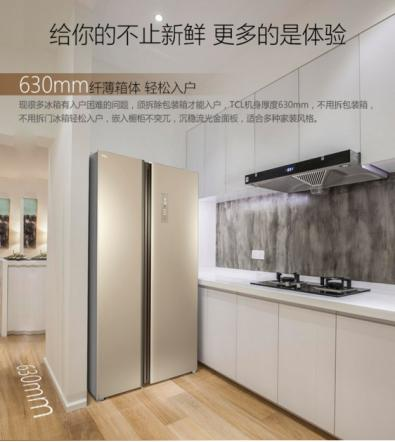清晰智能，尽显工匠精神

众所周知，TCL一直是中国电视制造领军品牌。在这次直播当中，单晓鹏给广大网友带来一款既好看又好玩的爆款人工智能电视单品TCL A950C。直播现场，当姗姗对着遥控器喊出“播放煎饼侠”时，电视立刻显示出《煎饼侠》的电影内容。TCL电视可快速而精准地实现搜索、播放和进度调控等功能，无愧智能之器，智慧之选。（袁姗姗用TCL人工智能电视语音搜索电影“煎饼侠”）

这款电视拥有航天级合金铝材，8.7mm金属窄边框设计，音响设计理念采用了高端电视最新流行的前置音响，前出音更宽阔。4000R曲率，更贴合人眼的弧度。4K+HDR新一代显示技术，能让4K画质呈现更清晰的明暗细节。在内容方面，和腾讯进行战略合作，具备海量优质影音资源，满足各种观看需求。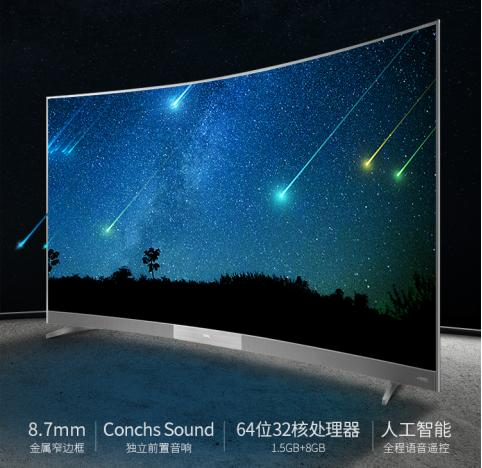除了以上环节之外，现场也有很多其他亮点故事，比如非常巧的是姗姗女神的人生第一部手机居然就是TCL当年的畅销款；TCL酷友科技CEO单晓鹏现场也跟大家分享了自己在海外的生活经历。大家如果有兴趣可以关注“TCL铁粉社区”官方微博，点击回看精彩直播视频。

一小时的直播很快就结束了，整场观看量十分可观，一直占据京东热门直播位置。这仅仅只是TCL2017“大咖价到”第一趴明星直播，接下来，在6月7日，作为TCL优生活匠心官的“水哥”王昱珩也会给大家带来同样精彩的直播，欢迎大家随时关注TCL官方微博平台获取“水哥”直播信息。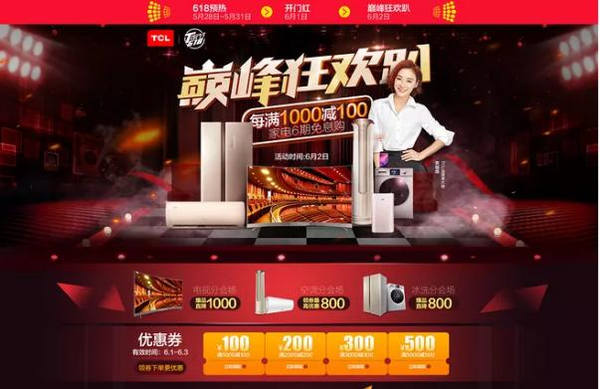此外，TCL京东专场的“巅峰狂欢趴”活动仍在继续，大家可登陆京东TCL品牌专区，了解更多优惠。TCL这次618大促是携品质新品全线参与，线上线下全平台让利互动，要想收获更多福利，赶紧关注TCL官方网站、京东、天猫和苏宁等平台TCL官方旗舰店。福利多多，等你来取。

`声明：本文由入驻焦点开放平台的作者撰写，除焦点官方账号外，观点仅代表作者本人，不代表焦点立场错误信息举报电话： 400-099-0099，邮箱：jubao@vip.sohu.com，或点此进行意见反馈，或点此进行举报投诉。`A B C D E F G H J K L M N P Q R S T W X Y Z
A - B - C - D - E
• A
• 鞍山
• 安庆
• 安阳
• 安顺
• 安康
• 澳门
• B
• 北京
• 保定
• 包头
• 巴彦淖尔
• 本溪
• 蚌埠
• 亳州
• 滨州
• 北海
• 百色
• 巴中
• 毕节
• 保山
• 宝鸡
• 白银
• 巴州
• C
• 承德
• 沧州
• 长治
• 赤峰
• 朝阳
• 长春
• 常州
• 滁州
• 池州
• 长沙
• 常德
• 郴州
• 潮州
• 崇左
• 重庆
• 成都
• 楚雄
• 昌都
• 慈溪
• 常熟
• D
• 大同
• 大连
• 丹东
• 大庆
• 东营
• 德州
• 东莞
• 德阳
• 达州
• 大理
• 德宏
• 定西
• 儋州
• 东平
• E
• 鄂尔多斯
• 鄂州
• 恩施
F - G - H - I - J
• F
• 抚顺
• 阜新
• 阜阳
• 福州
• 抚州
• 佛山
• 防城港
• G
• 赣州
• 广州
• 桂林
• 贵港
• 广元
• 广安
• 贵阳
• 固原
• H
• 邯郸
• 衡水
• 呼和浩特
• 呼伦贝尔
• 葫芦岛
• 哈尔滨
• 黑河
• 淮安
• 杭州
• 湖州
• 合肥
• 淮南
• 淮北
• 黄山
• 菏泽
• 鹤壁
• 黄石
• 黄冈
• 衡阳
• 怀化
• 惠州
• 河源
• 贺州
• 河池
• 海口
• 红河
• 汉中
• 海东
• 怀来
• I
• J
• 晋中
• 锦州
• 吉林
• 鸡西
• 佳木斯
• 嘉兴
• 金华
• 景德镇
• 九江
• 吉安
• 济南
• 济宁
• 焦作
• 荆门
• 荆州
• 江门
• 揭阳
• 金昌
• 酒泉
• 嘉峪关
K - L - M - N - P
• K
• 开封
• 昆明
• 昆山
• L
• 廊坊
• 临汾
• 辽阳
• 连云港
• 丽水
• 六安
• 龙岩
• 莱芜
• 临沂
• 聊城
• 洛阳
• 漯河
• 娄底
• 柳州
• 来宾
• 泸州
• 乐山
• 六盘水
• 丽江
• 临沧
• 拉萨
• 林芝
• 兰州
• 陇南
• M
• 牡丹江
• 马鞍山
• 茂名
• 梅州
• 绵阳
• 眉山
• N
• 南京
• 南通
• 宁波
• 南平
• 宁德
• 南昌
• 南阳
• 南宁
• 内江
• 南充
• P
• 盘锦
• 莆田
• 平顶山
• 濮阳
• 攀枝花
• 普洱
• 平凉
Q - R - S - T - W
• Q
• 秦皇岛
• 齐齐哈尔
• 衢州
• 泉州
• 青岛
• 清远
• 钦州
• 黔南
• 曲靖
• 庆阳
• R
• 日照
• 日喀则
• S
• 石家庄
• 沈阳
• 双鸭山
• 绥化
• 上海
• 苏州
• 宿迁
• 绍兴
• 宿州
• 三明
• 上饶
• 三门峡
• 商丘
• 十堰
• 随州
• 邵阳
• 韶关
• 深圳
• 汕头
• 汕尾
• 三亚
• 三沙
• 遂宁
• 山南
• 商洛
• 石嘴山
• T
• 天津
• 唐山
• 太原
• 通辽
• 铁岭
• 泰州
• 台州
• 铜陵
• 泰安
• 铜仁
• 铜川
• 天水
• 天门
• W
• 乌海
• 乌兰察布
• 无锡
• 温州
• 芜湖
• 潍坊
• 威海
• 武汉
• 梧州
• 渭南
• 武威
• 吴忠
• 乌鲁木齐
X - Y - Z
• X
• 邢台
• 徐州
• 宣城
• 厦门
• 新乡
• 许昌
• 信阳
• 襄阳
• 孝感
• 咸宁
• 湘潭
• 湘西
• 西双版纳
• 西安
• 咸阳
• 西宁
• 仙桃
• 西昌
• Y
• 运城
• 营口
• 盐城
• 扬州
• 鹰潭
• 宜春
• 烟台
• 宜昌
• 岳阳
• 益阳
• 永州
• 阳江
• 云浮
• 玉林
• 宜宾
• 雅安
• 玉溪
• 延安
• 榆林
• 银川
• Z
• 张家口
• 镇江
• 舟山
• 漳州
• 淄博
• 枣庄
• 郑州
• 周口
• 驻马店
• 株洲
• 张家界
• 珠海
• 湛江
• 肇庆
• 中山
• 自贡
• 资阳
• 遵义
• 昭通
• 张掖
• 中卫

1室1厅1厨1卫1阳台

1
2
3
4
5

0
1
2

1

1

0
1
2
3报名成功，资料已提交审核A B C D E F G H J K L M N P Q R S T W X Y Z
A - B - C - D - E
• A
• 鞍山
• 安庆
• 安阳
• 安顺
• 安康
• 澳门
• B
• 北京
• 保定
• 包头
• 巴彦淖尔
• 本溪
• 蚌埠
• 亳州
• 滨州
• 北海
• 百色
• 巴中
• 毕节
• 保山
• 宝鸡
• 白银
• 巴州
• C
• 承德
• 沧州
• 长治
• 赤峰
• 朝阳
• 长春
• 常州
• 滁州
• 池州
• 长沙
• 常德
• 郴州
• 潮州
• 崇左
• 重庆
• 成都
• 楚雄
• 昌都
• 慈溪
• 常熟
• D
• 大同
• 大连
• 丹东
• 大庆
• 东营
• 德州
• 东莞
• 德阳
• 达州
• 大理
• 德宏
• 定西
• 儋州
• 东平
• E
• 鄂尔多斯
• 鄂州
• 恩施
F - G - H - I - J
• F
• 抚顺
• 阜新
• 阜阳
• 福州
• 抚州
• 佛山
• 防城港
• G
• 赣州
• 广州
• 桂林
• 贵港
• 广元
• 广安
• 贵阳
• 固原
• H
• 邯郸
• 衡水
• 呼和浩特
• 呼伦贝尔
• 葫芦岛
• 哈尔滨
• 黑河
• 淮安
• 杭州
• 湖州
• 合肥
• 淮南
• 淮北
• 黄山
• 菏泽
• 鹤壁
• 黄石
• 黄冈
• 衡阳
• 怀化
• 惠州
• 河源
• 贺州
• 河池
• 海口
• 红河
• 汉中
• 海东
• 怀来
• I
• J
• 晋中
• 锦州
• 吉林
• 鸡西
• 佳木斯
• 嘉兴
• 金华
• 景德镇
• 九江
• 吉安
• 济南
• 济宁
• 焦作
• 荆门
• 荆州
• 江门
• 揭阳
• 金昌
• 酒泉
• 嘉峪关
K - L - M - N - P
• K
• 开封
• 昆明
• 昆山
• L
• 廊坊
• 临汾
• 辽阳
• 连云港
• 丽水
• 六安
• 龙岩
• 莱芜
• 临沂
• 聊城
• 洛阳
• 漯河
• 娄底
• 柳州
• 来宾
• 泸州
• 乐山
• 六盘水
• 丽江
• 临沧
• 拉萨
• 林芝
• 兰州
• 陇南
• M
• 牡丹江
• 马鞍山
• 茂名
• 梅州
• 绵阳
• 眉山
• N
• 南京
• 南通
• 宁波
• 南平
• 宁德
• 南昌
• 南阳
• 南宁
• 内江
• 南充
• P
• 盘锦
• 莆田
• 平顶山
• 濮阳
• 攀枝花
• 普洱
• 平凉
Q - R - S - T - W
• Q
• 秦皇岛
• 齐齐哈尔
• 衢州
• 泉州
• 青岛
• 清远
• 钦州
• 黔南
• 曲靖
• 庆阳
• R
• 日照
• 日喀则
• S
• 石家庄
• 沈阳
• 双鸭山
• 绥化
• 上海
• 苏州
• 宿迁
• 绍兴
• 宿州
• 三明
• 上饶
• 三门峡
• 商丘
• 十堰
• 随州
• 邵阳
• 韶关
• 深圳
• 汕头
• 汕尾
• 三亚
• 三沙
• 遂宁
• 山南
• 商洛
• 石嘴山
• T
• 天津
• 唐山
• 太原
• 通辽
• 铁岭
• 泰州
• 台州
• 铜陵
• 泰安
• 铜仁
• 铜川
• 天水
• 天门
• W
• 乌海
• 乌兰察布
• 无锡
• 温州
• 芜湖
• 潍坊
• 威海
• 武汉
• 梧州
• 渭南
• 武威
• 吴忠
• 乌鲁木齐
X - Y - Z
• X
• 邢台
• 徐州
• 宣城
• 厦门
• 新乡
• 许昌
• 信阳
• 襄阳
• 孝感
• 咸宁
• 湘潭
• 湘西
• 西双版纳
• 西安
• 咸阳
• 西宁
• 仙桃
• 西昌
• Y
• 运城
• 营口
• 盐城
• 扬州
• 鹰潭
• 宜春
• 烟台
• 宜昌
• 岳阳
• 益阳
• 永州
• 阳江
• 云浮
• 玉林
• 宜宾
• 雅安
• 玉溪
• 延安
• 榆林
• 银川
• Z
• 张家口
• 镇江
• 舟山
• 漳州
• 淄博
• 枣庄
• 郑州
• 周口
• 驻马店
• 株洲
• 张家界
• 珠海
• 湛江
• 肇庆
• 中山
• 自贡
• 资阳
• 遵义
• 昭通
• 张掖
• 中卫• 手机• 分享
• 设计
免费设计
• 计算器
装修计算器
• 入驻
合作入驻
• 联系
联系我们
• 置顶
返回顶部